In :
%matplotlib inline
import pandas as pd
import numpy as np
import matplotlib.pyplot as plt
import warnings
from pandas.core.common import SettingWithCopyWarning
warnings.simplefilter("ignore", SettingWithCopyWarning)

from IPython.core.display import HTML
def css_styling():
return HTML(styles)
css_styling()

Out:

# A Primer on Bayesian Methods for Multilevel Modeling¶

Hierarchical or multilevel modeling is a generalization of regression modeling.

Multilevel models are regression models in which the constituent model parameters are given probability models. This implies that model parameters are allowed to vary by group.

Observational units are often naturally clustered. Clustering induces dependence between observations, despite random sampling of clusters and random sampling within clusters.

A hierarchical model is a particular multilevel model where parameters are nested within one another.

Some multilevel structures are not hierarchical.

• e.g. "country" and "year" are not nested, but may represent separate, but overlapping, clusters of parameters

We will motivate this topic using an environmental epidemiology example.

### Example: Radon contamination (Gelman and Hill 2006)¶

Radon is a radioactive gas that enters homes through contact points with the ground. It is a carcinogen that is the primary cause of lung cancer in non-smokers. Radon levels vary greatly from household to household.The EPA did a study of radon levels in 80,000 houses. Two important predictors:

• measurement in basement or first floor (radon higher in basements)
• county uranium level (positive correlation with radon levels)

We will focus on modeling radon levels in Minnesota.

The hierarchy in this example is households within county.

### Data organization¶

First, we import the data from a local file, and extract Minnesota's data.

In :
# Import radon data
srrs2.columns = srrs2.columns.map(str.strip)
srrs_mn = srrs2[srrs2.state=='MN']


Next, obtain the county-level predictor, uranium, by combining two variables.

In :
srrs_mn['fips'] = srrs_mn.stfips*1000 + srrs_mn.cntyfips
cty_mn = cty[cty.st=='MN']
cty_mn['fips'] = 1000*cty_mn.stfips + cty_mn.ctfips


Use the merge method to combine home- and county-level information in a single DataFrame.

In :
srrs_mn = srrs_mn.merge(cty_mn[['fips', 'Uppm']], on='fips')
srrs_mn = srrs_mn.drop_duplicates(subset='idnum')
u = np.log(srrs_mn.Uppm)

n = len(srrs_mn)


We also need a lookup table (dict) for each unique county, for indexing.

In :
srrs_mn.county = srrs_mn.county.map(str.strip)
mn_counties = srrs_mn.county.unique()
county_lookup = dict(zip(mn_counties, range(len(mn_counties))))


Finally, create local copies of variables.

In :
county = srrs_mn['county_code'] = srrs_mn.county.replace(county_lookup).values
floor_measure = srrs_mn.floor.values


Distribution of radon levels in MN (log scale):

In :
srrs_mn.activity.apply(lambda x: np.log(x+0.1)).hist(bins=25)

Out:
<matplotlib.axes._subplots.AxesSubplot at 0x109fa77f0>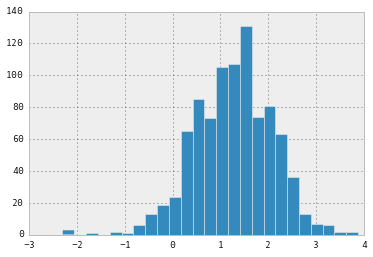## Conventional approaches¶

The two conventional alternatives to modeling radon exposure represent the two extremes of the bias-variance tradeoff:

Complete pooling:

Treat all counties the same, and estimate a single radon level.

$$y_i = \alpha + \beta x_i + \epsilon_i$$

No pooling:

Model radon in each county independently.

$$y_i = \alpha_{j[i]} + \beta x_i + \epsilon_i$$

where $j = 1,\ldots,85$

The errors $\epsilon_i$ may represent measurement error, temporal within-house variation, or variation among houses.

Here are the point estimates of the slope and intercept for the complete pooling model:

In :
from pymc3 import glm, Model, Metropolis, NUTS, sample

with Model() as pooled_model:
pooled_trace = sample(1000, NUTS())

/Users/fonnescj/GitHub/notebook/src/ipython/IPython/html.py:14: ShimWarning: The IPython.html package has been deprecated. You should import from notebook instead. IPython.html.widgets has moved to ipywidgets.
"IPython.html.widgets has moved to ipywidgets.", ShimWarning)
/Users/fonnescj/GitHub/notebook/src/ipython/IPython/utils/traitlets.py:5: UserWarning: IPython.utils.traitlets has moved to a top-level traitlets package.
warn("IPython.utils.traitlets has moved to a top-level traitlets package.")
/usr/local/Cellar/python3/3.4.2_1/Frameworks/Python.framework/Versions/3.4/lib/python3.4/importlib/_bootstrap.py:321: RuntimeWarning: numpy.ndarray size changed, may indicate binary incompatibility
return f(*args, **kwds)

 [-----------------100%-----------------] 1000 of 1000 complete in 2.2 sec
In :
b0, m0 = pooled_trace['Intercept'][500:].mean(), pooled_trace['floor'][500:].mean()

In :
plt.scatter(srrs_mn.floor, np.log(srrs_mn.activity+0.1))
xvals = np.linspace(-0.2, 1.2)
plt.plot(xvals, m0*xvals+b0, 'r--')

Out:
[<matplotlib.lines.Line2D at 0x10fb08470>]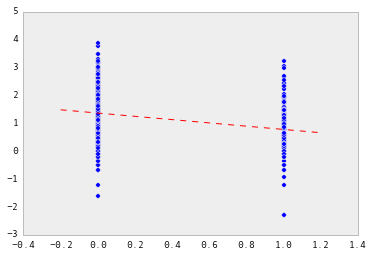Estimates of county radon levels for the unpooled model:

In :
from statsmodels.formula.api import ols
unpooled_fit = ols('log_radon ~ C(county) + floor - 1', srrs_mn).fit()
unpooled_estimates = unpooled_fit.params

In :
unpooled_estimates = unpooled_estimates.rename(dict(zip(unpooled_estimates.index.values,
[x[10:-1] or x for x in unpooled_estimates.index.values])))
unpooled_se = unpooled_fit.HC3_se.rename(dict(zip(unpooled_fit.HC3_se.index.values,
[x[10:-1] or x for x in unpooled_fit.HC3_se.index.values])))


We can plot the ordered estimates to identify counties with high radon levels:

In :
order = unpooled_estimates.order()[1:].index

plt.scatter(range(len(unpooled_estimates)-1), unpooled_estimates[order])
for i, m, se in zip(range(len(unpooled_estimates)-1), unpooled_estimates[order], unpooled_se[order]):
plt.plot([i,i], [m-se, m+se], 'b-')
plt.xlim(-1,86); plt.ylim(-1,4)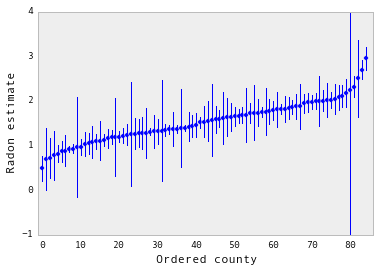Here are visual comparisons between the pooled and unpooled estimates for a subset of counties representing a range of sample sizes.

In :
sample_counties = ('LAC QUI PARLE', 'AITKIN', 'KOOCHICHING',
'DOUGLAS', 'CLAY', 'STEARNS', 'RAMSEY', 'ST LOUIS')

fig, axes = plt.subplots(2, 4, figsize=(12, 6), sharey=True, sharex=True)
axes = axes.ravel()
for i,c in enumerate(sample_counties):
x = srrs_mn.floor[srrs_mn.county==c]
axes[i].scatter(x + np.random.randn(len(x))*0.01, y, alpha=0.4)

# No pooling model
m,b = unpooled_estimates[['floor', c]]

# Plot both models and data
xvals = np.linspace(-0.2, 1.2)
axes[i].plot(xvals, m*xvals+b)
axes[i].plot(xvals, m0*xvals+b0, 'r--')
axes[i].set_xticks([0,1])
axes[i].set_xticklabels(['basement', 'floor'])
axes[i].set_ylim(-1, 3)
axes[i].set_title(c)
if not i%2: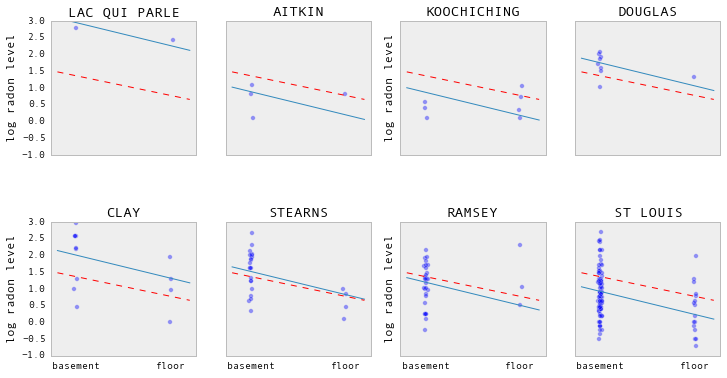Neither of these models are satisfactory:

• if we are trying to identify high-radon counties, pooling is useless
• we do not trust extreme unpooled estimates produced by models using few observations

## Multilevel and hierarchical models¶

When we pool our data, we imply that they are sampled from the same model. This ignores any variation among sampling units (other than sampling variance):When we analyze data unpooled, we imply that they are sampled independently from separate models. At the opposite extreme from the pooled case, this approach claims that differences between sampling units are to large to combine them:In a hierarchical model, parameters are viewed as a sample from a population distribution of parameters. Thus, we view them as being neither entirely different or exactly the same. This is parital pooling.## PyMC 3¶We can use PyMC to easily specify multilevel models, and fit them using Markov chain Monte Carlo.

Features:

• Fits Bayesian statistical models with Markov chain Monte Carlo and other algorithms.
• Includes a large suite of well-documented statistical distributions.
• Uses NumPy and Theano for fast numerical computation.
• Creates posterior summaries including tables and plots.
• Several convergence diagnostics and goodness-of-fit procedures are available.
• Extensible: easily incorporates custom MCMC algorithms and unusual probability distributions.
• MCMC loops can be embedded in larger programs, and results can be analyzed with the full power of Python.

## Partial pooling model¶

The simplest partial pooling model for the household radon dataset is one which simply estimates radon levels, without any predictors at any level. A partial pooling model represents a compromise between the pooled and unpooled extremes, approximately a weighted average (based on sample size) of the unpooled county estimates and the pooled estimates.

$$\hat{\alpha} \approx \frac{(n_j/\sigma_y^2)\bar{y}_j + (1/\sigma_{\alpha}^2)\bar{y}}{(n_j/\sigma_y^2) + (1/\sigma_{\alpha}^2)}$$

Estimates for counties with smaller sample sizes will shrink towards the state-wide average.

Estimates for counties with larger sample sizes will be closer to the unpooled county estimates.

In :
from pymc3 import Normal, Model, Uniform

with Model() as partial_pooling:

# Priors
mu_a = Normal('mu_a', mu=0., tau=0.0001)
sigma_a = Uniform('sigma_a', lower=0, upper=100)
tau_a = sigma_a**-2

# Random intercepts
a = Normal('a', mu=mu_a, tau=tau_a, shape=len(set(county)))

# Model error
sigma_y = Uniform('sigma_y', lower=0, upper=100)
tau_y = sigma_y**-2

# Expected value
y_hat = a[county]

# Data likelihood
y_like = Normal('y_like', mu=y_hat, tau=tau_y, observed=log_radon)

In :
from pymc3 import sample

with partial_pooling:

step = NUTS()

partial_pooling_samples = sample(2000, step)

 [-----------------100%-----------------] 2000 of 2000 complete in 7.9 sec

Notice the difference between the unpooled and partially-pooled estimates, particularly at smaller sample sizes. The former are both more extreme and more imprecise.

In :
sample_trace = partial_pooling_samples['a'][-1000:]

fig, axes = plt.subplots(1, 2, figsize=(14,6), sharex=True, sharey=True)
samples, counties = sample_trace.shape
jitter = np.random.normal(scale=0.1, size=counties)

n_county = srrs_mn.groupby('county')['idnum'].count()
unpooled = pd.DataFrame({'n':n_county, 'm':unpooled_means, 'sd':unpooled_sd})
unpooled['se'] = unpooled.sd/np.sqrt(unpooled.n)

axes.plot(unpooled.n + jitter, unpooled.m, 'b.')
for j, row in zip(jitter, unpooled.iterrows()):
name, dat = row
axes.plot([dat.n+j,dat.n+j], [dat.m-dat.se, dat.m+dat.se], 'b-')
axes.set_xscale('log')
axes.hlines(sample_trace.mean(), 0.9, 100, linestyles='--')

samples, counties = sample_trace.shape
means = sample_trace.mean(axis=0)
sd = sample_trace.std(axis=0)
axes.scatter(n_county.values + jitter, means)
axes.set_xscale('log')
axes.set_xlim(1,100)
axes.set_ylim(0, 3)
axes.hlines(sample_trace.mean(), 0.9, 100, linestyles='--')
for j,n,m,s in zip(jitter, n_county.values, means, sd):
s.sort()
axes.plot([n+j]*2, [m-s, m+s], 'b-')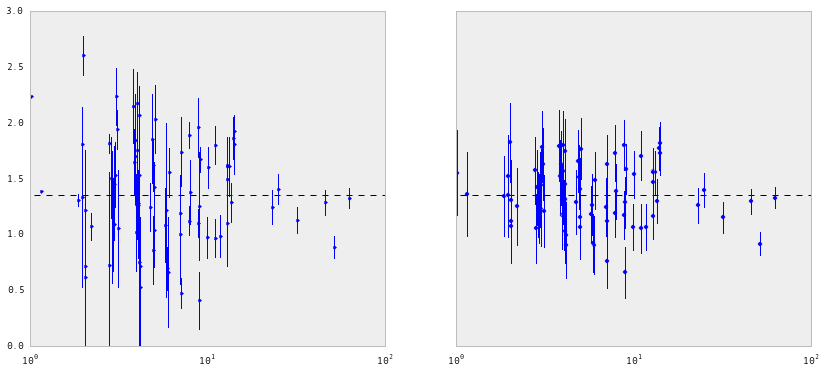## Varying intercept model¶

This model allows intercepts to vary across county, according to a random effect.

$$y_i = \alpha_{j[i]} + \beta x_{i} + \epsilon_i$$

where

$$\epsilon_i \sim N(0, \sigma_y^2)$$

and the intercept random effect:

$$\alpha_{j[i]} \sim N(\mu_{\alpha}, \sigma_{\alpha}^2)$$

As with the the “no-pooling” model, we set a separate intercept for each county, but rather than fitting separate least squares regression models for each county, multilevel modeling shares strength among counties, allowing for more reasonable inference in counties with little data.

In :
with Model() as varying_intercept:

# Priors
mu_a = Normal('mu_a', mu=0., tau=0.0001)
sigma_a = Uniform('sigma_a', lower=0, upper=100)
tau_a = sigma_a**-2

# Random intercepts
a = Normal('a', mu=mu_a, tau=tau_a, shape=len(set(county)))
# Common slope
b = Normal('b', mu=0., tau=0.0001)

# Model error
sigma_y = Uniform('sigma_y', lower=0, upper=100)
tau_y = sigma_y**-2

# Expected value
y_hat = a[county] + b * floor_measure

# Data likelihood
y_like = Normal('y_like', mu=y_hat, tau=tau_y, observed=log_radon)


We can fit the above model using MCMC. Here, we use the No U-turn Sampler (NUTS), which is a gradient-based method for MCMC simulation.

In :
with varying_intercept:

step = NUTS()

varying_intercept_samples = sample(2000, step)

 [-----------------100%-----------------] 2000 of 2000 complete in 6.1 sec
In :
from pymc3 import forestplot

plt.figure(figsize=(6,10))
forestplot(varying_intercept_samples, vars=['a'])

Out:
<matplotlib.gridspec.GridSpec at 0x117163668>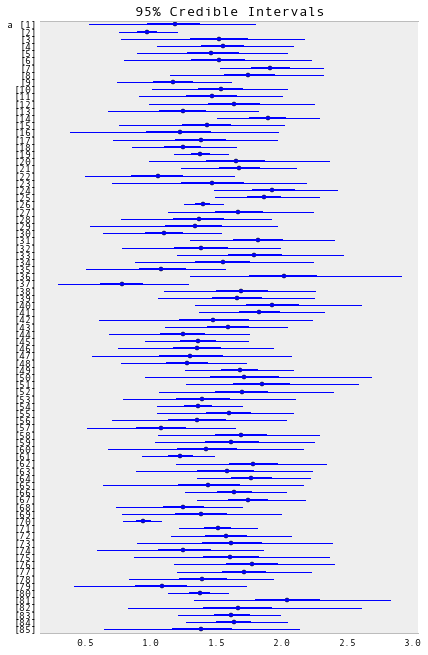In :
from pymc3 import traceplot

traceplot(varying_intercept_samples[-1000:], vars=['sigma_a', 'b'])

Out:
array([[<matplotlib.axes._subplots.AxesSubplot object at 0x11771b6d8>,
<matplotlib.axes._subplots.AxesSubplot object at 0x1177f5208>],
[<matplotlib.axes._subplots.AxesSubplot object at 0x11785d630>,
<matplotlib.axes._subplots.AxesSubplot object at 0x117896cf8>]], dtype=object)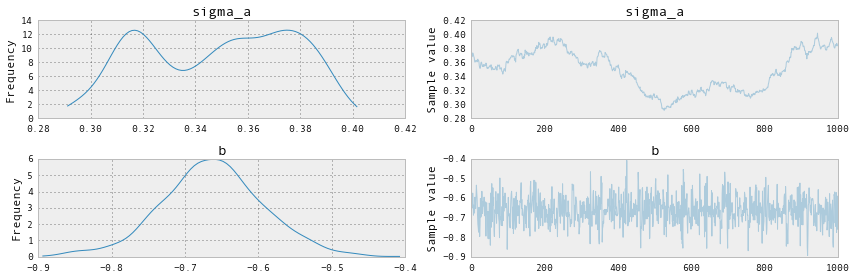The estimate for the floor coefficient is approximately -0.66, which can be interpreted as houses without basements having about half ($\exp(-0.66) = 0.52$) the radon levels of those with basements, after accounting for county.

In :
from pymc3 import summary

summary(varying_intercept_samples[-1000:], vars=['b'])

b:

Mean             SD               MC Error         95% HPD interval
-------------------------------------------------------------------

-0.662           0.070            0.002            [-0.798, -0.519]

Posterior quantiles:
2.5            25             50             75             97.5
|--------------|==============|==============|--------------|

-0.807         -0.706         -0.662         -0.619         -0.523


In :
xvals = np.arange(2)
bp = varying_intercept_samples['a'].mean(axis=0)
mp = varying_intercept_samples['b'].mean()
for bi in bp:
plt.plot(xvals, mp*xvals + bi, 'bo-', alpha=0.4)
plt.xlim(-0.1,1.1);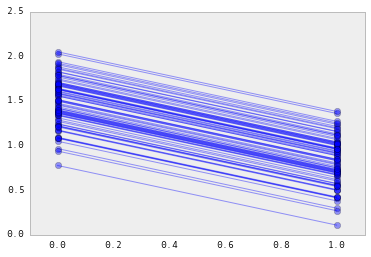It is easy to show that the partial pooling model provides more objectively reasonable estimates than either the pooled or unpooled models, at least for counties with small sample sizes.

In :
fig, axes = plt.subplots(2, 4, figsize=(12, 6), sharey=True, sharex=True)
axes = axes.ravel()
for i,c in enumerate(sample_counties):

# Plot county data
x = srrs_mn.floor[srrs_mn.county==c]
axes[i].scatter(x + np.random.randn(len(x))*0.01, y, alpha=0.4)

# No pooling model
m,b = unpooled_estimates[['floor', c]]

xvals = np.linspace(-0.2, 1.2)
# Unpooled estimate
axes[i].plot(xvals, m*xvals+b)
# Pooled estimate
axes[i].plot(xvals, m0*xvals+b0, 'r--')
# Partial pooling esimate
axes[i].plot(xvals, mp*xvals+bp[county_lookup[c]], 'k:')
axes[i].set_xticks([0,1])
axes[i].set_xticklabels(['basement', 'floor'])
axes[i].set_ylim(-1, 3)
axes[i].set_title(c)
if not i%2: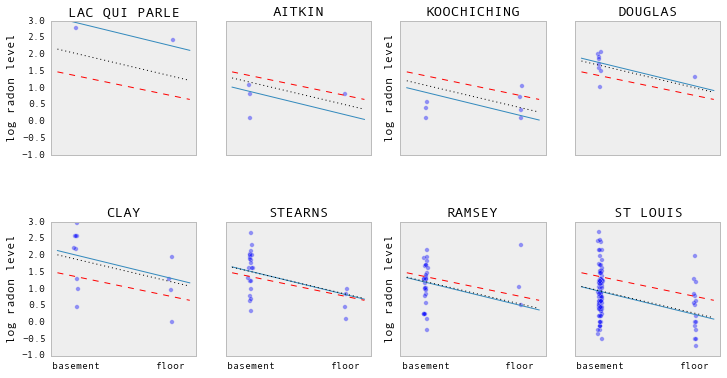## Varying slope model¶

Alternatively, we can posit a model that allows the counties to vary according to how the location of measurement (basement or floor) influences the radon reading.

$$y_i = \alpha + \beta_{j[i]} x_{i} + \epsilon_i$$
In :
with Model() as varying_slope:

# Priors
mu_b = Normal('mu_b', mu=0., tau=0.0001)
sigma_b = Uniform('sigma_b', lower=0, upper=100)
tau_b = sigma_b**-2

# Model intercept
a = Normal('a', mu=0., tau=0.0001)
# Random slopes
b = Normal('b', mu=mu_b, tau=tau_b, shape=len(set(county)))

# Model error
sigma_y = Uniform('sigma_y', lower=0, upper=100)
tau_y = sigma_y**-2

# Expected value
y_hat = a + b[county] * floor_measure

# Data likelihood
y_like = Normal('y_like', mu=y_hat, tau=tau_y, observed=log_radon)

In :
with varying_slope:

step = NUTS()

varying_slope_samples = sample(2000, step)

 [-----------------100%-----------------] 2000 of 2000 complete in 7.6 sec
In :
plt.figure(figsize=(6,10))
forestplot(varying_slope_samples, vars=['b'])

Out:
<matplotlib.gridspec.GridSpec at 0x11a0994a8>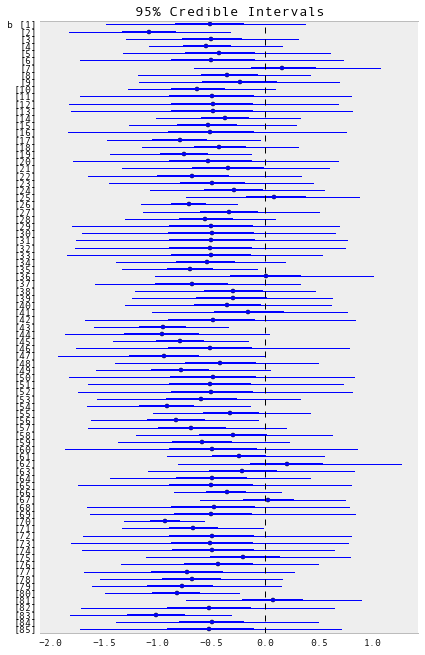In :
xvals = np.arange(2)
b = varying_slope_samples['a'].mean()
m = varying_slope_samples['b'].mean(axis=0)
for mi in m:
plt.plot(xvals, mi*xvals + b, 'bo-', alpha=0.4)
plt.xlim(-0.2, 1.2);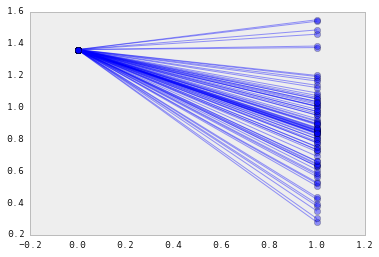## Varying intercept and slope model¶

The most general model allows both the intercept and slope to vary by county:

$$y_i = \alpha_{j[i]} + \beta_{j[i]} x_{i} + \epsilon_i$$
In :
with Model() as varying_intercept_slope:

# Priors
mu_a = Normal('mu_a', mu=0., tau=0.0001)
sigma_a = Uniform('sigma_a', lower=0, upper=100)
tau_a = sigma_a**-2

mu_b = Normal('mu_b', mu=0., tau=0.0001)
sigma_b = Uniform('sigma_b', lower=0, upper=100)
tau_b = sigma_b**-2

# Random intercepts
a = Normal('a', mu=mu_a, tau=tau_a, shape=len(set(county)))
# Random slopes
b = Normal('b', mu=mu_b, tau=tau_b, shape=len(set(county)))

# Model error
sigma_y = Uniform('sigma_y', lower=0, upper=100)
tau_y = sigma_y**-2

# Expected value
y_hat = a[county] + b[county] * floor_measure

# Data likelihood
y_like = Normal('y_like', mu=y_hat, tau=tau_y, observed=log_radon)

In :
with varying_intercept_slope:

step = NUTS()

both_varying_samples = sample(2000, step)

 [-----------------100%-----------------] 2000 of 2000 complete in 9.5 sec
In :
plt.figure(figsize=(6,16))
forestplot(both_varying_samples, vars=['a','b'])

Out:
<matplotlib.gridspec.GridSpec at 0x11e9e97f0>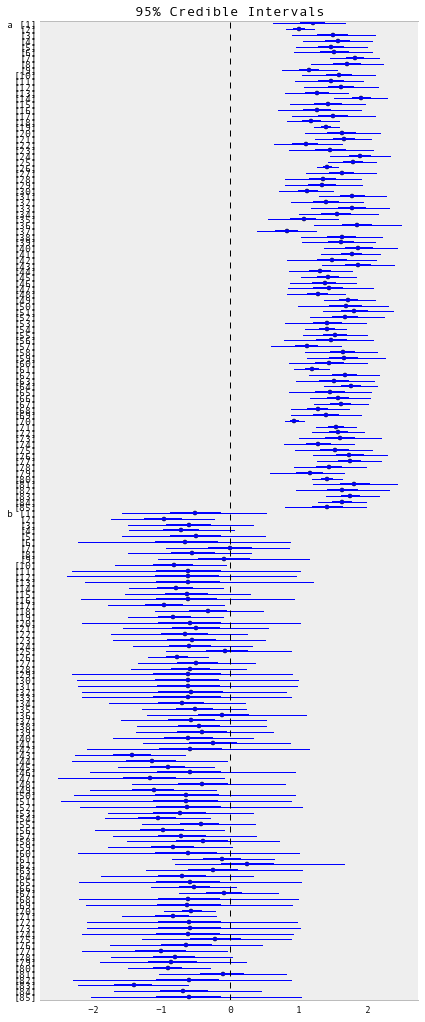In :
xvals = np.arange(2)
b = both_varying_samples['a'].mean(axis=0)
m = both_varying_samples['b'].mean(axis=0)
for bi,mi in zip(b,m):
plt.plot(xvals, mi*xvals + bi, 'bo-', alpha=0.4)
plt.xlim(-0.1, 1.1);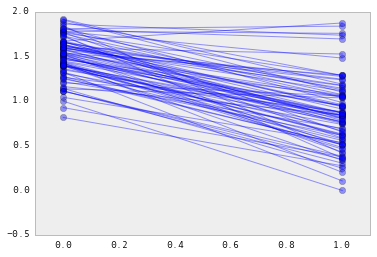A primary strength of multilevel models is the ability to handle predictors on multiple levels simultaneously. If we consider the varying-intercepts model above:

$$y_i = \alpha_{j[i]} + \beta x_{i} + \epsilon_i$$

we may, instead of a simple random effect to describe variation in the expected radon value, specify another regression model with a county-level covariate. Here, we use the county uranium reading $u_j$, which is thought to be related to radon levels:

$$\alpha_j = \gamma_0 + \gamma_1 u_j + \zeta_j$$$$\zeta_j \sim N(0, \sigma_{\alpha}^2)$$

Thus, we are now incorporating a house-level predictor (floor or basement) as well as a county-level predictor (uranium).

Note that the model has both indicator variables for each county, plus a county-level covariate. In classical regression, this would result in collinearity. In a multilevel model, the partial pooling of the intercepts towards the expected value of the group-level linear model avoids this.

Group-level predictors also serve to reduce group-level variation $\sigma_{\alpha}$. An important implication of this is that the group-level estimate induces stronger pooling.

In :
from pymc3 import Deterministic

with Model() as hierarchical_intercept:

# Priors
sigma_a = Uniform('sigma_a', lower=0, upper=100)
tau_a = sigma_a**-2

# County uranium model for slope
gamma_0 = Normal('gamma_0', mu=0., tau=0.0001)
gamma_1 = Normal('gamma_1', mu=0., tau=0.0001)

# Uranium model for intercept
mu_a = gamma_0 + gamma_1*u
# County variation not explained by uranium
eps_a = Normal('eps_a', mu=0, tau=tau_a, shape=len(set(county)))
a = Deterministic('a', mu_a + eps_a[county])

# Random slope
b = Normal('b', mu=0, tau=0.001)

# Model error
sigma_y = Uniform('sigma_y', lower=0, upper=100)
tau_y = sigma_y**-2

# Expected value
y_hat = a + b * floor_measure

# Data likelihood
y_like = Normal('y_like', mu=y_hat, tau=tau_y, observed=log_radon)

In :
with hierarchical_intercept:

step = NUTS()

hierarchical_samples = sample(2000, step)

 [-----------------100%-----------------] 2000 of 2000 complete in 7.8 sec
In :
trace = hierarchical_samples[-1000:]
a_means = trace['a'].mean(axis=0)
plt.scatter(u, a_means)
g0 = trace['gamma_0'].mean()
g1 = trace['gamma_1'].mean()
xvals = np.linspace(-1, 0.8)
plt.plot(xvals, g0+g1*xvals, 'k--')
plt.xlim(-1, 0.8)

a_se = trace['a'].std(axis=0)
for ui, m, se in zip(u, a_means, a_se):
plt.plot([ui,ui], [m-se, m+se], 'b-')
plt.xlabel('County-level uranium'); plt.ylabel('Intercept estimate')

Out:
<matplotlib.text.Text at 0x12007d9b0>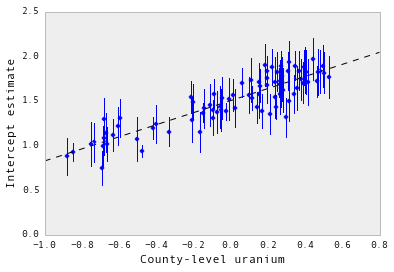The standard errors on the intercepts are narrower than for the partial-pooling model without a county-level covariate.

### Correlations among levels¶

In some instances, having predictors at multiple levels can reveal correlation between individual-level variables and group residuals. We can account for this by including the average of the individual predictors as a covariate in the model for the group intercept.

$$\alpha_j = \gamma_0 + \gamma_1 u_j + \gamma_2 \bar{x} + \zeta_j$$

These are broadly referred to as contextual effects.

In :
# Create new variable for mean of floor across counties
xbar = srrs_mn.groupby('county')['floor'].mean().rename(county_lookup)

In :
with Model() as contextual_effect:

# Priors
sigma_a = Uniform('sigma_a', lower=0, upper=100)
tau_a = sigma_a**-2

# County uranium model for slope
gamma_0 = Normal('gamma_0', mu=0., tau=0.0001)
gamma_1 = Normal('gamma_1', mu=0., tau=0.0001)
gamma_2 = Normal('gamma_2', mu=0., tau=0.0001)

# Uranium model for intercept
mu_a = gamma_0 + gamma_1*u + gamma_2*xbar[county]

# County variation not explained by uranium
eps_a = Normal('eps_a', mu=0, tau=tau_a, shape=len(set(county)))
a = Deterministic('a', mu_a + eps_a[county])

# Random slope
b = Normal('b', mu=0, tau=0.001)

# Model error
sigma_y = Uniform('sigma_y', lower=0, upper=100)
tau_y = sigma_y**-2

# Expected value
y_hat = a + b * floor_measure

# Data likelihood
y_like = Normal('y_like', mu=y_hat, tau=tau_y, observed=log_radon)

In :
with contextual_effect:

step = NUTS()

contextual_samples = sample(2000, step)

 [-----------------100%-----------------] 2000 of 2000 complete in 7.0 sec
In :
traceplot(contextual_samples[-1000:], vars=['gamma_2'])

Out:
array([[<matplotlib.axes._subplots.AxesSubplot object at 0x11d39be10>,
<matplotlib.axes._subplots.AxesSubplot object at 0x120a8ccf8>]], dtype=object)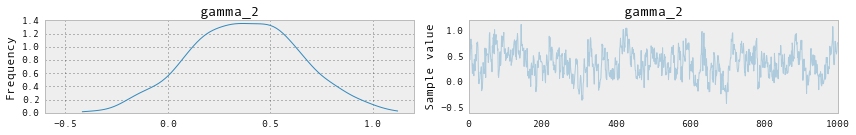In :
summary(contextual_samples[-1000:], vars=['gamma_2'])

gamma_2:

Mean             SD               MC Error         95% HPD interval
-------------------------------------------------------------------

0.375            0.266            0.020            [-0.190, 0.846]

Posterior quantiles:
2.5            25             50             75             97.5
|--------------|==============|==============|--------------|

-0.144         0.188          0.378          0.558          0.907



So, we might infer from this that counties with higher proportions of houses without basements tend to have higher baseline levels of radon. Perhaps this is related to the soil type, which in turn might influence what type of structures are built.

### Prediction¶

Gelman (2006) used cross-validation tests to check the prediction error of the unpooled, pooled, and partially-pooled models

root mean squared cross-validation prediction errors:

• unpooled = 0.86
• pooled = 0.84
• multilevel = 0.79

There are two types of prediction that can be made in a multilevel model:

1. a new individual within an existing group
2. a new individual within a new group

For example, if we wanted to make a prediction for a new house with no basement in St. Louis county, we just need to sample from the radon model with the appropriate intercept.

In :
county_lookup['ST LOUIS']

Out:
69

That is,

$$\tilde{y}_i \sim N(\alpha_{69} + \beta (x_i=1), \sigma_y^2$$

This is simply a matter of adding a single additional line in PyMC:

In :
with Model() as prediction:

# Priors
sigma_a = Uniform('sigma_a', lower=0, upper=100)
tau_a = sigma_a**-2

# County uranium model for slope
gamma_0 = Normal('gamma_0', mu=0., tau=0.0001)
gamma_1 = Normal('gamma_1', mu=0., tau=0.0001)
gamma_2 = Normal('gamma_2', mu=0., tau=0.0001)

# Uranium model for intercept
mu_a = gamma_0 + gamma_1*u + gamma_2*xbar[county]

# County variation not explained by uranium
eps_a = Normal('eps_a', mu=0, tau=tau_a, shape=len(set(county)))
a = Deterministic('a', mu_a + eps_a[county])

# Random slope
b = Normal('b', mu=0, tau=0.001)

# Model error
sigma_y = Uniform('sigma_y', lower=0, upper=100)
tau_y = sigma_y**-2

# Expected value
y_hat = a + b * floor_measure

# Data likelihood
y_like = Normal('y_like', mu=y_hat, tau=tau_y, observed=log_radon)

# St Louis county prediction
yhat_stl = Normal('yhat_stl', mu=a + b, tau=tau_y)

In [ ]:
with prediction:

step = NUTS()

prediction_sample = sample(2000, step)

In [ ]:
summary(prediction_sample[-1000:], vars=['yhat_stl'])


## Benefits of Multilevel Models¶

Accounting for natural hierarchical structure of observational data

Estimation of coefficients for (under-represented) groups

Incorporating individual- and group-level information when estimating group-level coefficients

Allowing for variation among individual-level coefficients across groups

1. Gelman, A., & Hill, J. (2006). Data Analysis Using Regression and Multilevel/Hierarchical Models (1st ed.). Cambridge University Press.

2. Gelman, A. (2006). Multilevel (Hierarchical) modeling: what it can and cannot do. Technometrics, 48(3), 432–435.